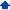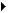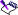### Performing matrix operations

A matrix is a rectangular array of numbers. Each column in a matrix corresponds to one variable, and each row corresponds to a linear constraint. Quattro Pro lets you multiply two matrices and invert a matrix.

Matrix operations can help you solve sets of linear formulas and equations. For example, suppose you have these four unique equations using variables w, x, y, and z:

1w+1x+2y+3z=10

3w+2x+2y+1z=20

1w+0x+3y+4z=15

1w+1x+0y+1z=6

You can express the coefficients (the numbers multiplying the variables w, x, y, and z) of these equations in this “4 by 4″ coefficient matrix:

1 1 2 3

3 2 2 1

1 0 3 4

1 1 0 1

And you can express the results of the equations in this “4 by 1″ constant matrix (the first variable is at the top of the list, the second is next, and so on):

10

20

15

6

You can use matrix multiplication and inversion to find the value of each variable in the second matrix that satisfies the constraints in the first matrix.

Matrix multiplication is helpful when you need to multiply the elements of one matrix by the elements of a second and sum their products. For example, matrix multiplication can calculate the cost of several types of building materials across development phases, or it can compute job costs involving different labor categories.

Matrix inversion is the first step in solving sets of linear equations. When you multiply a matrix by its inverse, the resultant matrix is an identity matrix (all 1s and 0s, with only a single diagonal of 1s). You cannot invert a matrix that is mathematically singular. A matrix is singular when its determinant equals zero. You cannot invert a matrix with mathematical redundancies or inconsistencies. You can invert “square” matrices only. A square matrix is one with the same number of rows and columns.

#### To multiply two matrices1 Click ToolsNumeric toolsMultiply matrices.
 2 Click the Range pickerin the Matrix 1 box, and select the first matrix to multiply.
 3 Click the Range pickerin the Matrix 2 box, and select the second matrix to multiply.
 4 Click the Range pickerin the Destination box, and specify the destination cells.• The number of columns in the first matrix must equal the number of rows in the second matrix.

#### To invert a matrix1 Click ToolsNumeric toolsInvert.
 2 Click the Range pickerin the Source box, and select the matrix cells to invert.
 3 Click the Range pickerin the Destination box, and select the upper left cell of the cells where you want to write the inverted matrix.• You can invert matrices of sizes up to 90 rows by 90 columns.
 • If you specify the same source cells as the destination cells, the inverted matrix overwrites the existing matrix.